### What is Wiris Quizzes?

Wiris Quizzes is an assessment tool with computer-based grading that enhances the usual standard question types in UR Courses with mathematical and scientific functionalities.

How to add wiris quiz question types in UR Courses?

1. Create quiz activity, if you dont know how to create a quiz please refer to how to add a quiz to your course page.
2. Once the quiz has been created.

• Click edit quiz button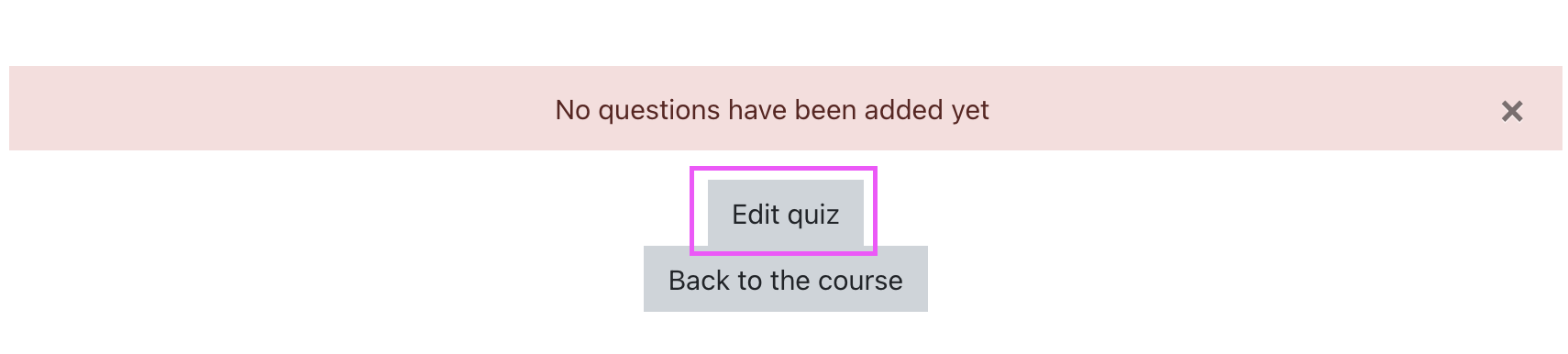• Or Click actions Menu button or the three gear icon on the top right corner of the quiz and click edit quiz button as highlighted on the image below.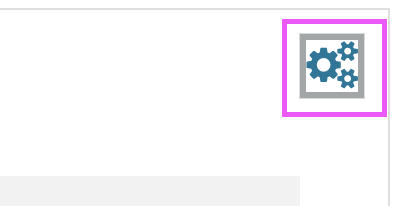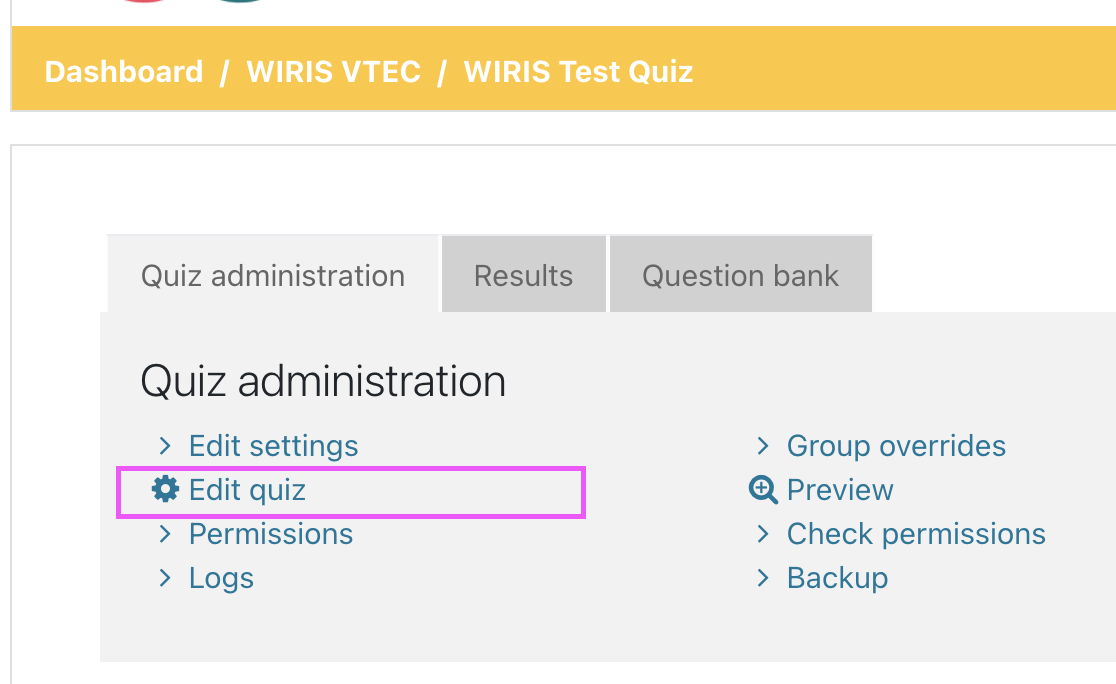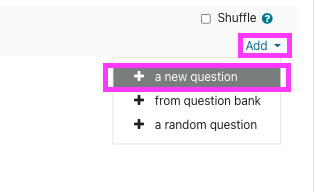• Choose question type popup window will open, scroll down to the bottom of the popup window and the Wiris question types should be presented as highlighted on the image below.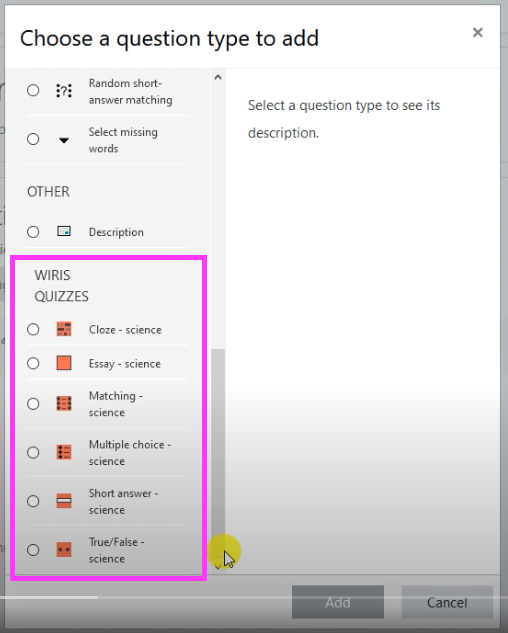### WIRIS variables

In WIRIS, variable are defined with # in each beginning part of variable that being used in each question.

• Defining a Variables

For example: See Essay question for your reference
Find the value of x?
5 - x = 2 will be written as #a - x = #ans

• Defining a Random variables in the Algorithm window
a = random(1,5)
ans = random(1,10)

Here is the example of this question

### MathType and ChemType Interface

MathType is a mathematical notation created by WIRIS that is integraded witihin UR Courses. MathType allows you to write notation(equations/formulas/expressions etc.) from the most simpliest to the most complex notation that you can used for each of your questions.

ChemType is a flavor of MathType designed to help you work with chemical notation. A personalized toolbar with the common chemical symbols but also a different user experience adapted to chemical notation needs.

To open MathType interface simply expand your text editor and find the squareroot symbol as shown in the image below.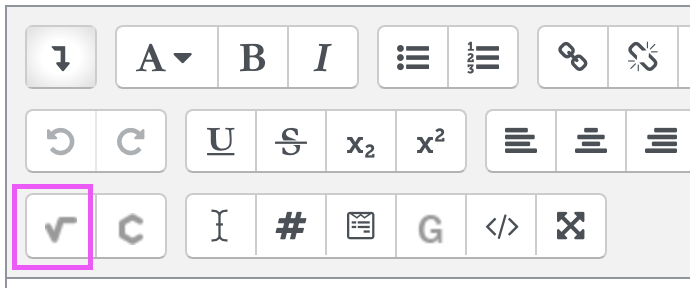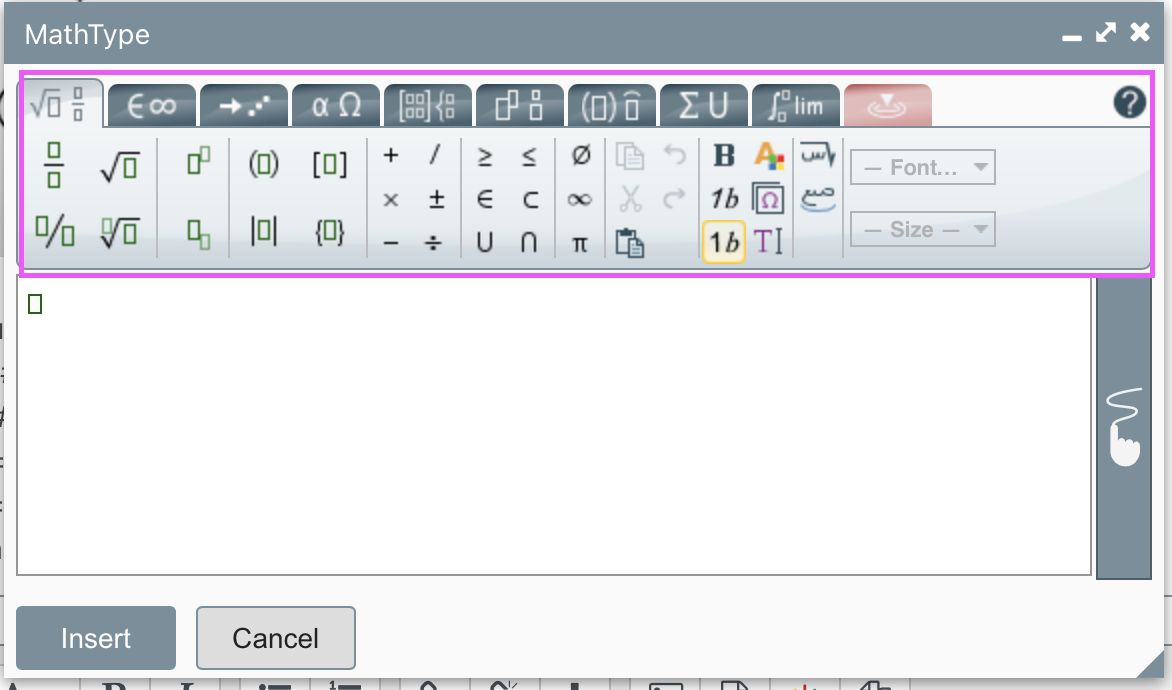Here is a full tutorial on how to use MathType provided by WIRIS

Other useful examples provided by WIRIS

To open ChemType interface simply expand your text editor and find the chemistry symbol as shown in the image below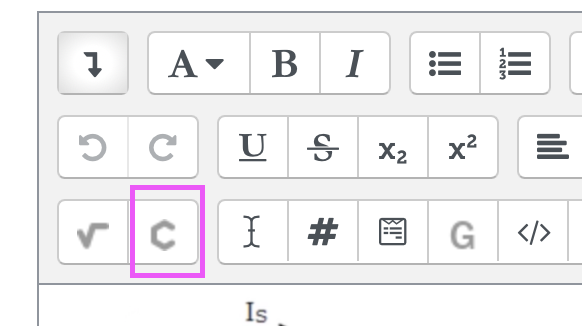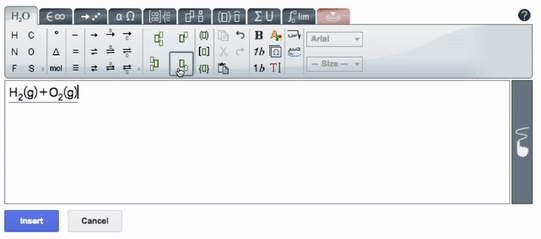Here is a full documentation of ChemType provided by WIRIS

### Question types

In this section, we list the WIRIS question types and describe how they work.

WIRIS question types has the same setup as the stadard UR Courses question. The advantage of Using WIRIS questions is the ability of a Teacher to enhances the usual standard UR Courses questions with mathematical and scientific functionalities.

### Essay

Ussually students answer this question type by writing long answer inside a text editor.

In WIRIS Essay question type, you have now the ability to insert formulas using the MathType button, defining random variables and using them in the question text. You only need to write the question's statement and the algorithm of CalcMe.

This question type does not have automatic grading. The teachers must review the answers and manually grade them – students will not have a complete quiz grade until then.

One simple way to setup WIRIS Essay question:

Fill in the required metadata as you can see in the mage below.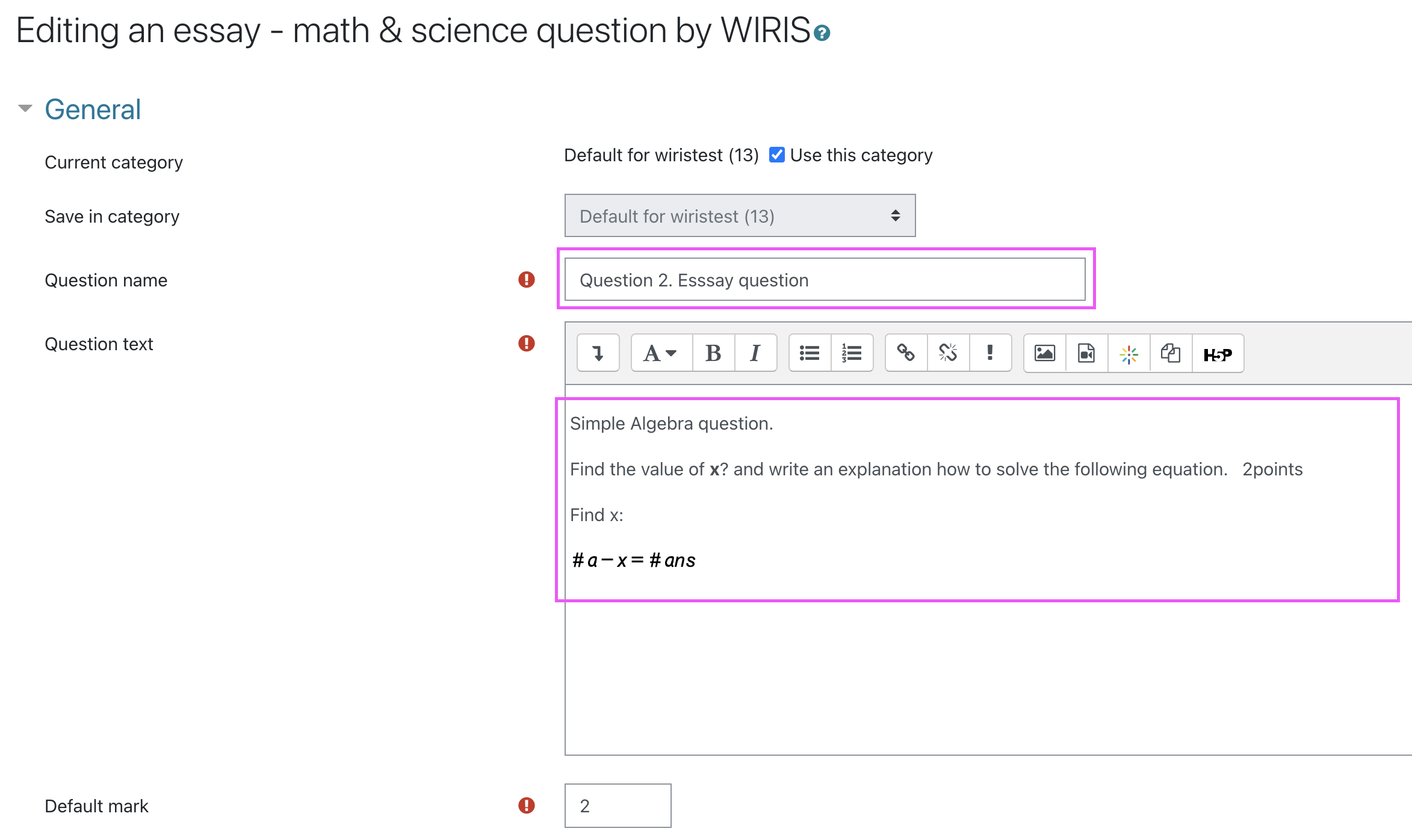Essay question: Find the value of x? and write an explanation how to solve the following equation.

5 - x = 2

In this example we simply defined the variable as #a - x = #ans and we want that the numbers to be randomly changing when a student view the question. In order for us to achieve that set up we need to open the algorithm settings for this question.

In the quiz settings goto the WIRIS variable option then click the gear icon to open the WIRISQuizzes Studio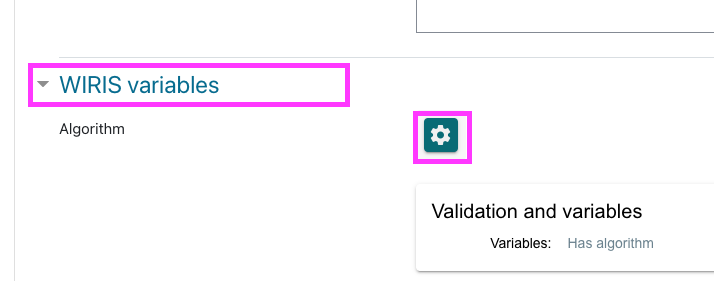### WIRISQuizzes Studio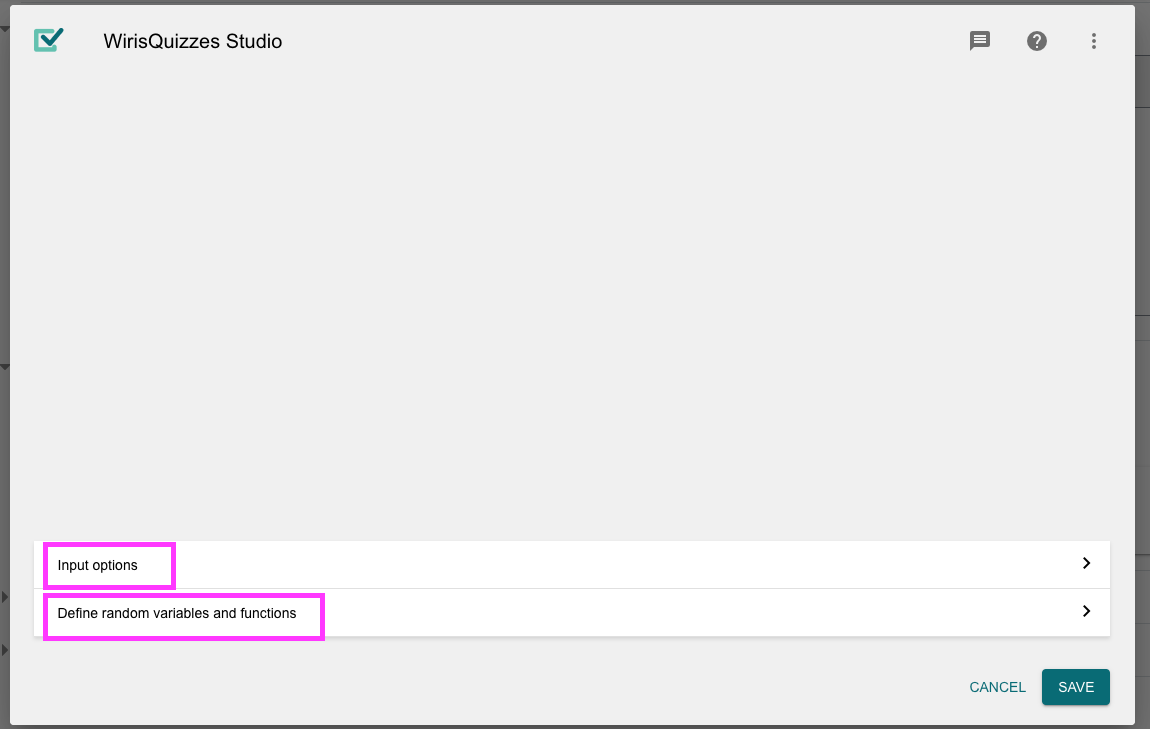### Input options

Here, you can choose what kind of auxiliary entry you want to offer to the students. In Essay question there are four options: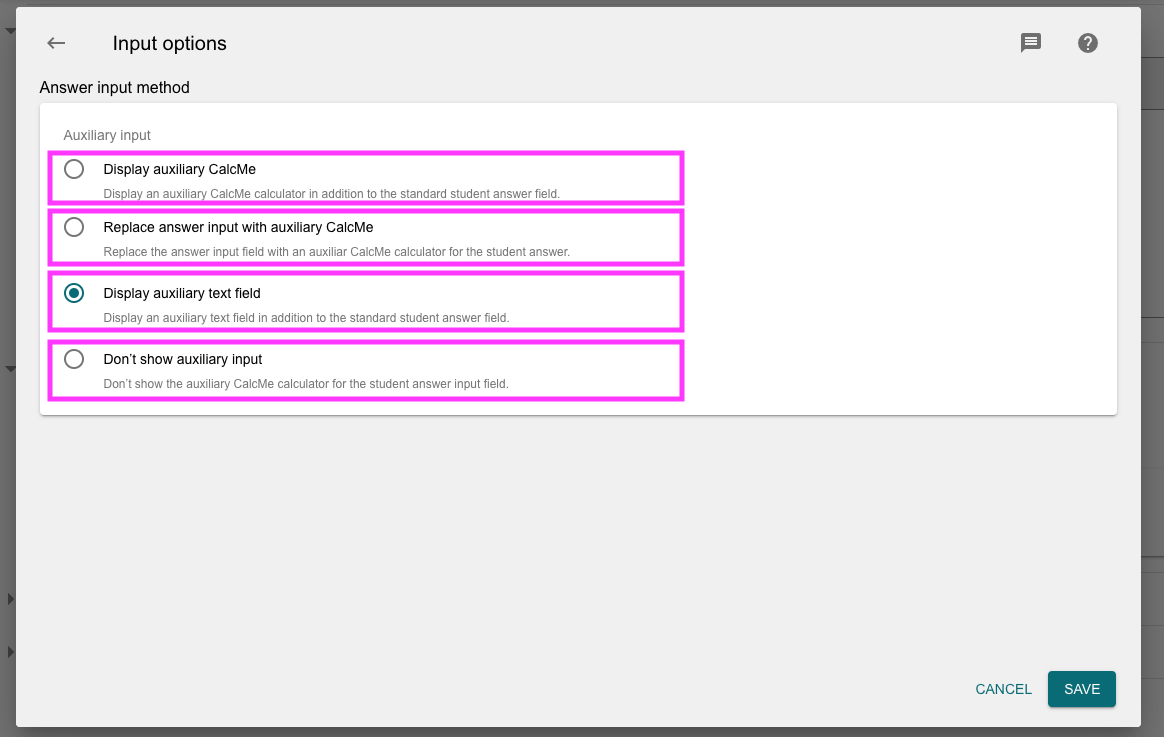### Define random variables and functions

We will be using the code editor(algorithm button) to group our variables and functions.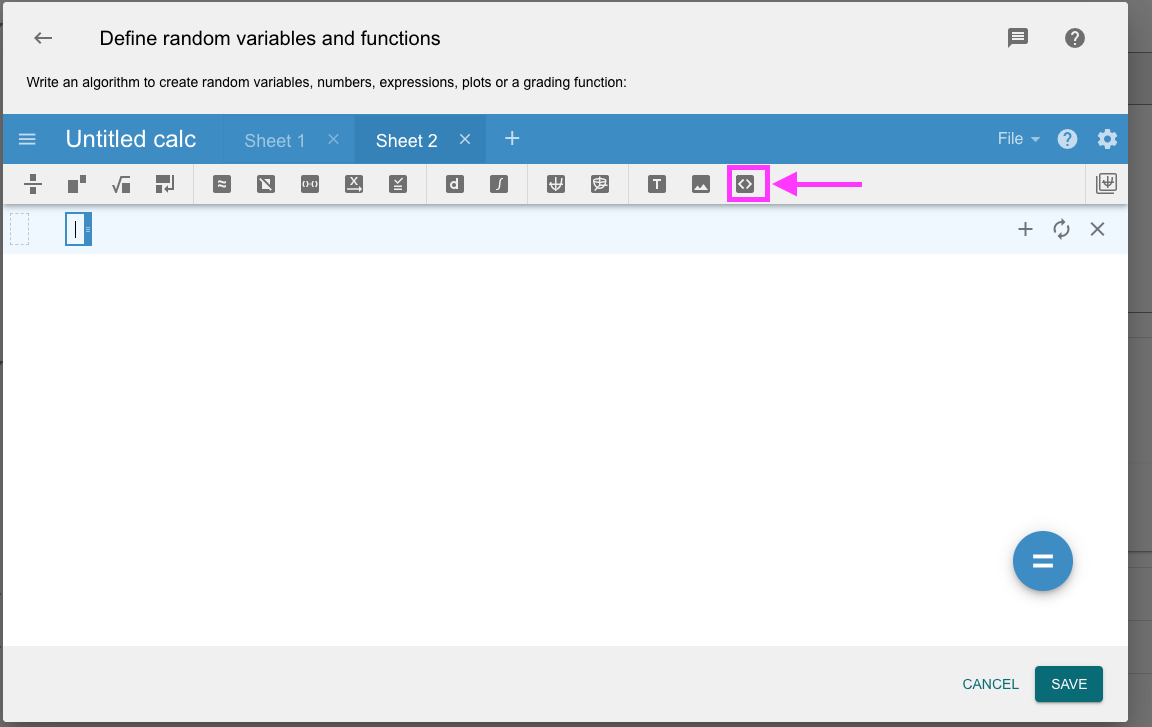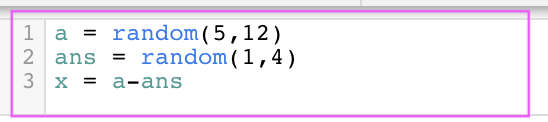To define a random variable we assign

a = random(5,12) - will be equal to a = [5,6,7,8,9,10,11,12] and the value of a will be changing as the student preview the question.

ans = random(1,4) - will be equal to ans = [1,2,3,4] and the value of ans will be changing as the student preview the question.

x = a-ans this is the formula to get the of value x

### Testing

In WIRIS you will be able to test your variable and formulas if they are correctly calculating.

To run the test simply click on the CalcMe editor then enter the variables that you want to get their value.

In this example we will be entering variable a then hit return/enter on the keyboard or click on the bottom of the variable or click the + sign to add new entry.

Next will be variable ans then variable x

Then click the = sign on the calme editor to calculate and display the results.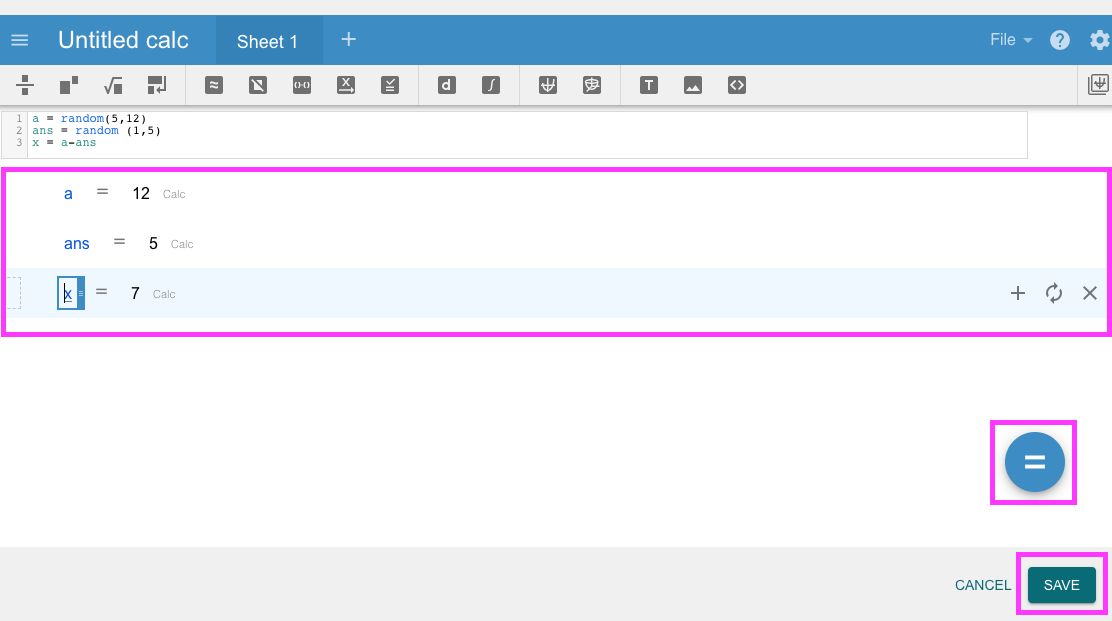Dont forget to hit save button.

Students answer this question type by writing a number or short formula, using MathType. This is the most complex question type to make because the student can write an answer in several different ways, and the system must decide if they are correct. That's why this question type has more sections to configure it.

In particular, there is a section called Validation options, which handles the way in which Wiris Quizzes interprets formulas from the student.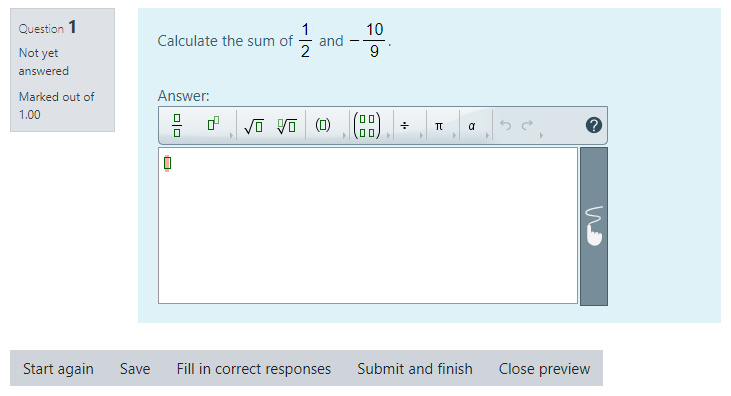From the student's point of view, this type is just a special type of Short Answer question, but with multiple questions and answers combined into one.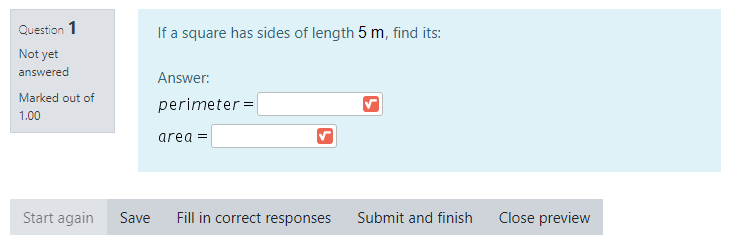In the menu of Create a new question... there is no Compound answer type. Choose Short answer question.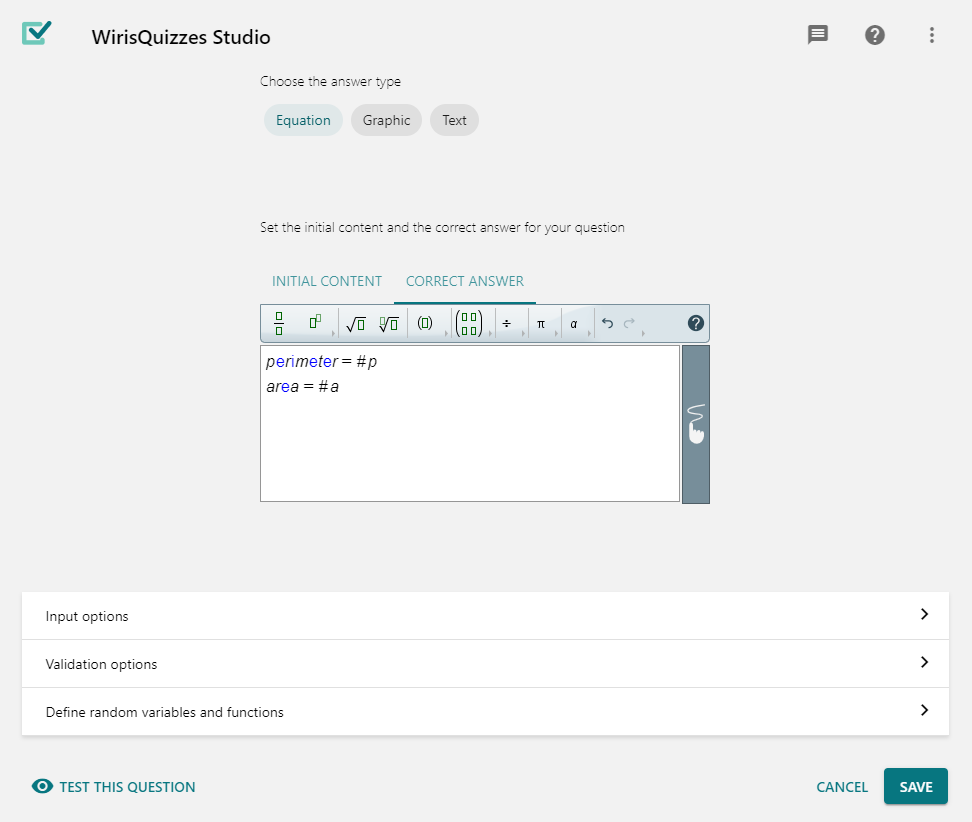From the Input options section, set the Grade answer boxes separately checkbox in the Compound answer blank. Then you can list the sub-questions in the Correct answer field, using the form question = answer, one per line. The question is just a label and can be any text. The answer can be any valid Short answer correct answer, including numbers, formulas, and #variables

You can set the grading of each sub-question. Choose if all sub-answers must be correct, or you want different weights for each sub-answer.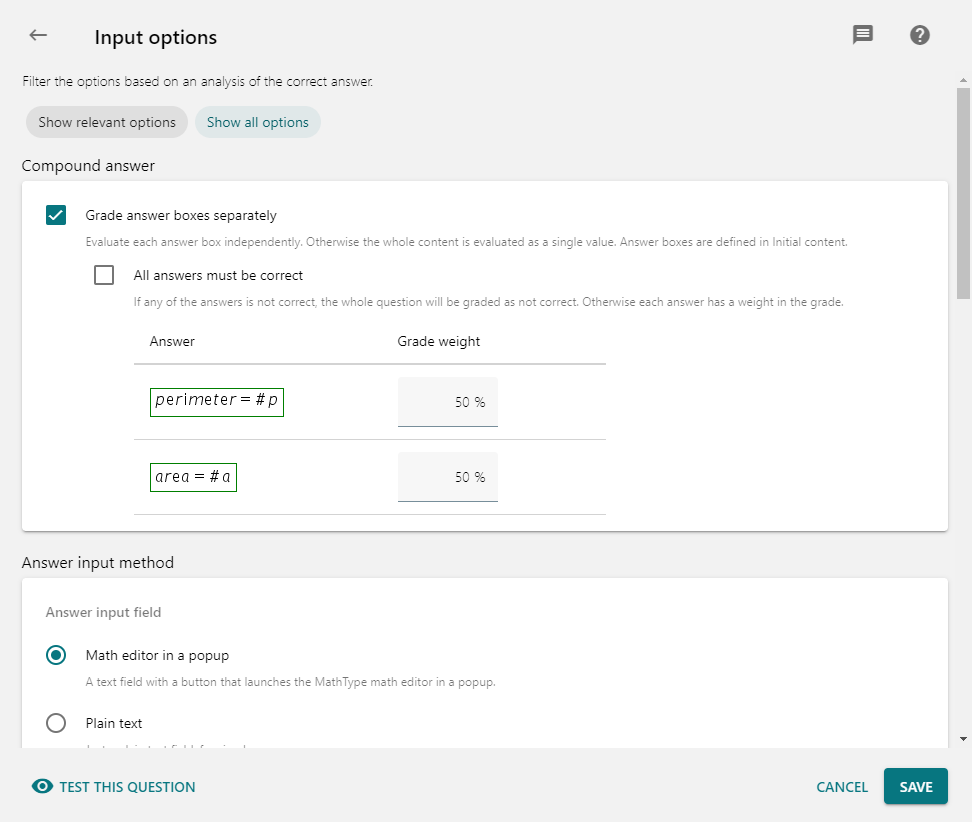### Multiple choice

Students answer this question type just choosing one (or maybe many) options from a list.

Note Moodle now allows questions to have negative scoring, so now you can set grades in a way to discourage answering at random. But keep in mind some web browsers do not allow to deselect a choice once you have selected it.

As always, you can define variables and use them in the Question text and Feedback. In this question type, they can also be used in the answer choices.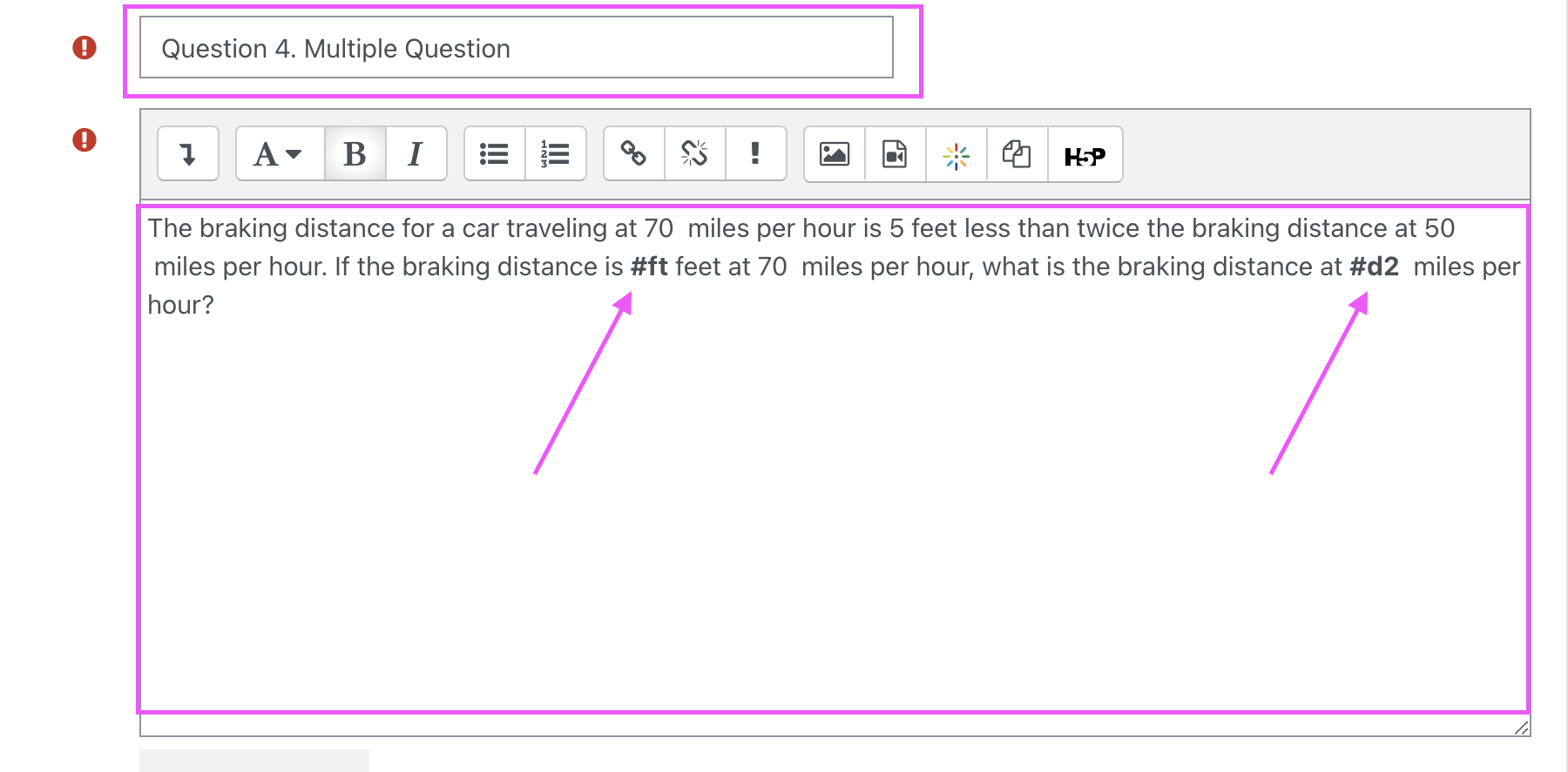To create the example above we will create a (random) variable ft, and d2 and give three wrong answers also in random. For convenience, we will set the first answer as the correct choice but don't worry, the system will automatically shuffle the choices to the student.

This will be the correct answer.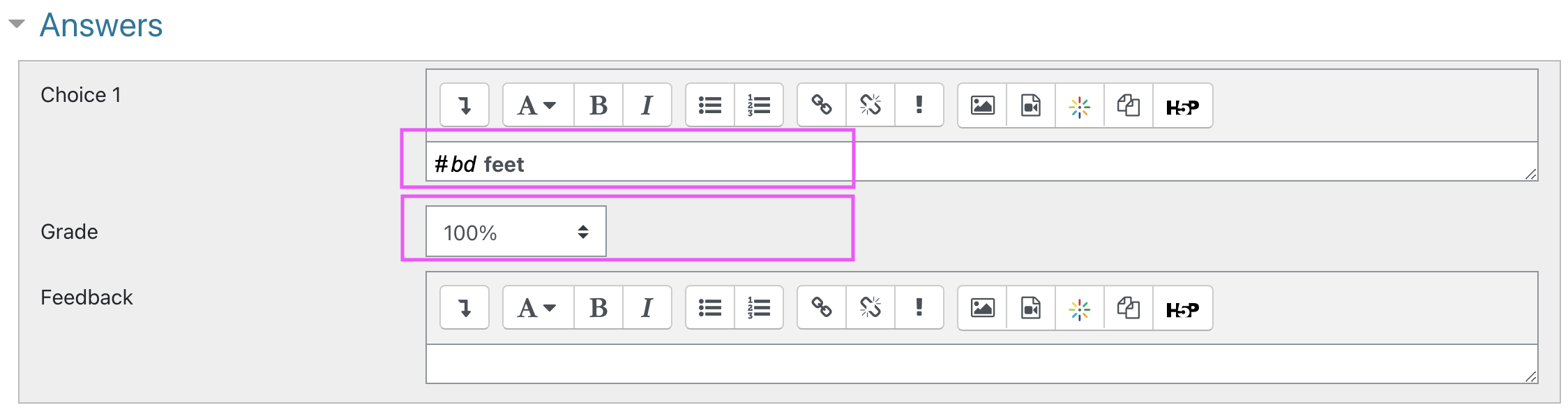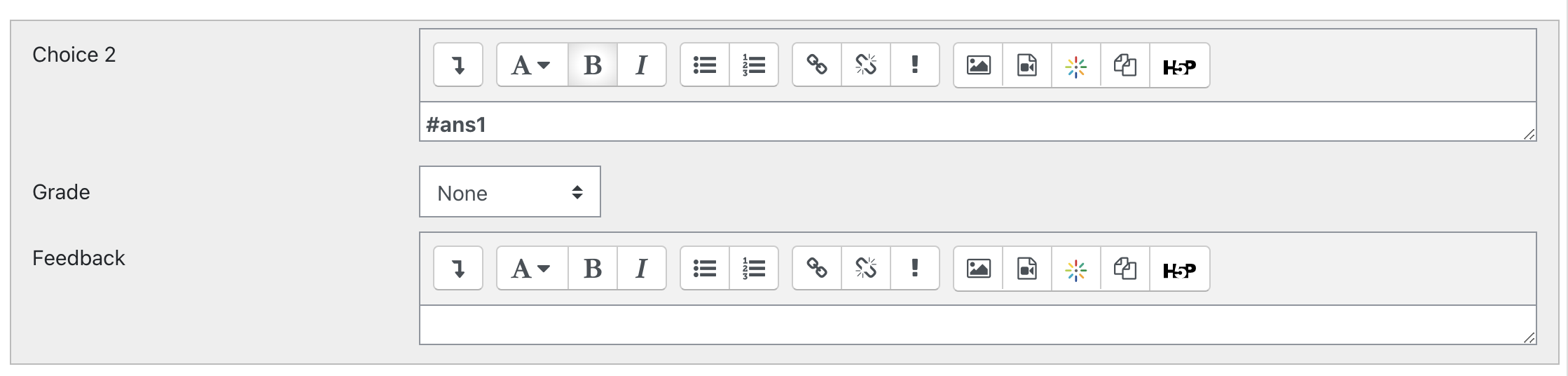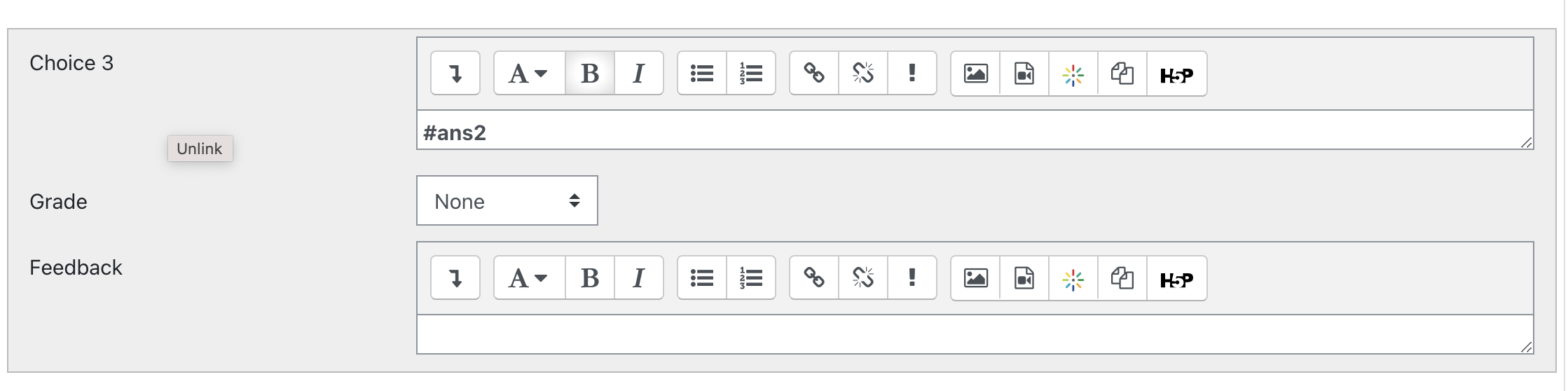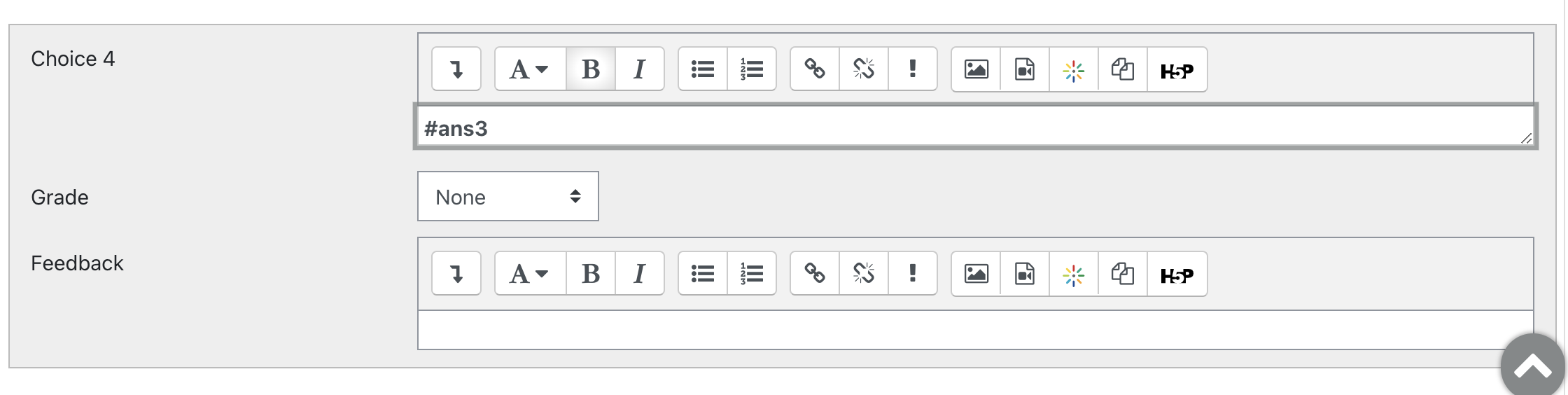If you preview the question and answers now you can see that the font face of the answers are different from the defined WIRIS variable.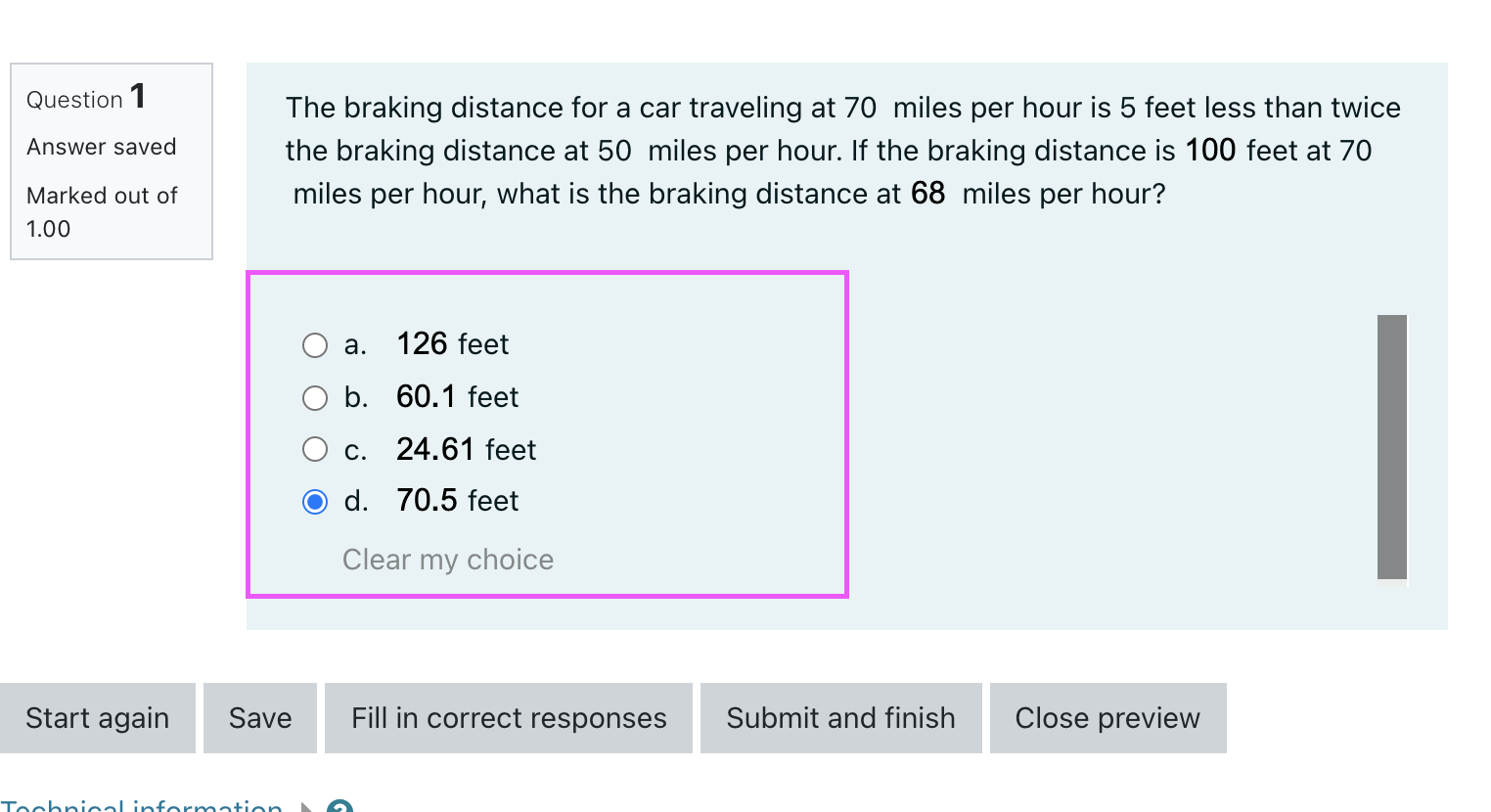In the quiz settings goto the WIRIS variable option then click the gear icon to open the WIRISQuizzes Studio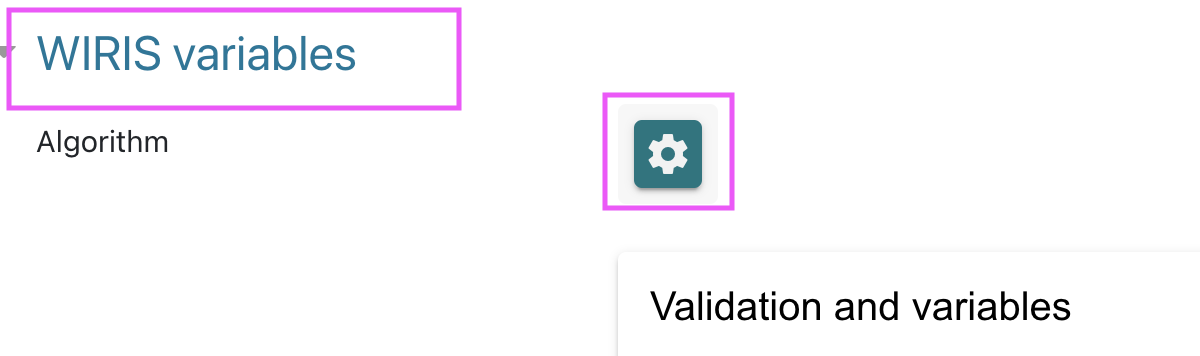Then repeat the steps on question above on how to select your inputs, define your varible, formulas and function.

### The algorithm would be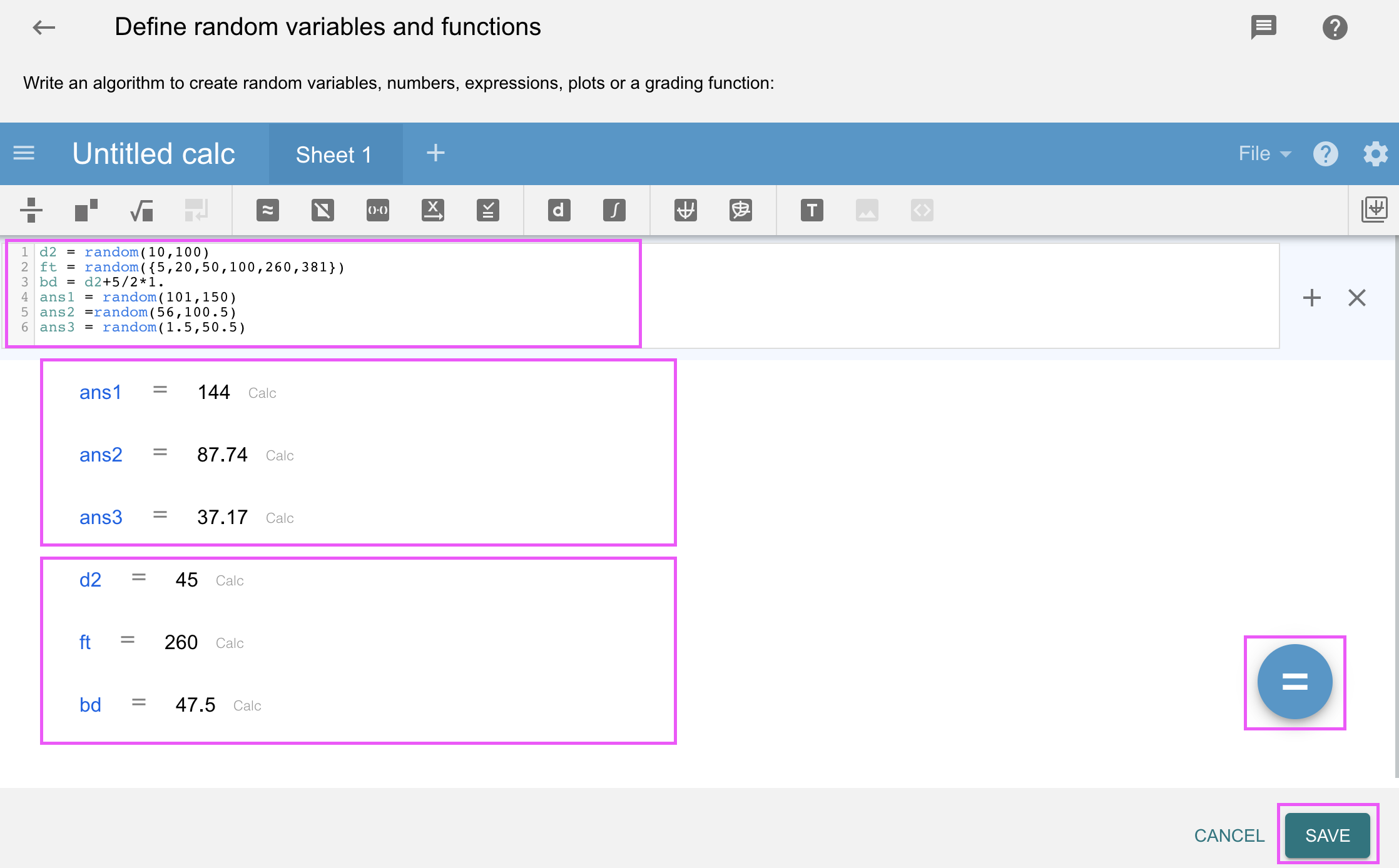### Matching

Students answer this question type by choosing a simple label for each item on a list.

You can still use variables in the Answers, but their values must be texts, numbers, or simple formulas. On the other hand, questions can be anything, notably complex formulas or even plots.

Write the algorithm to define the variables you will use in the Question text and Feedback, and more importantly in questions and answers.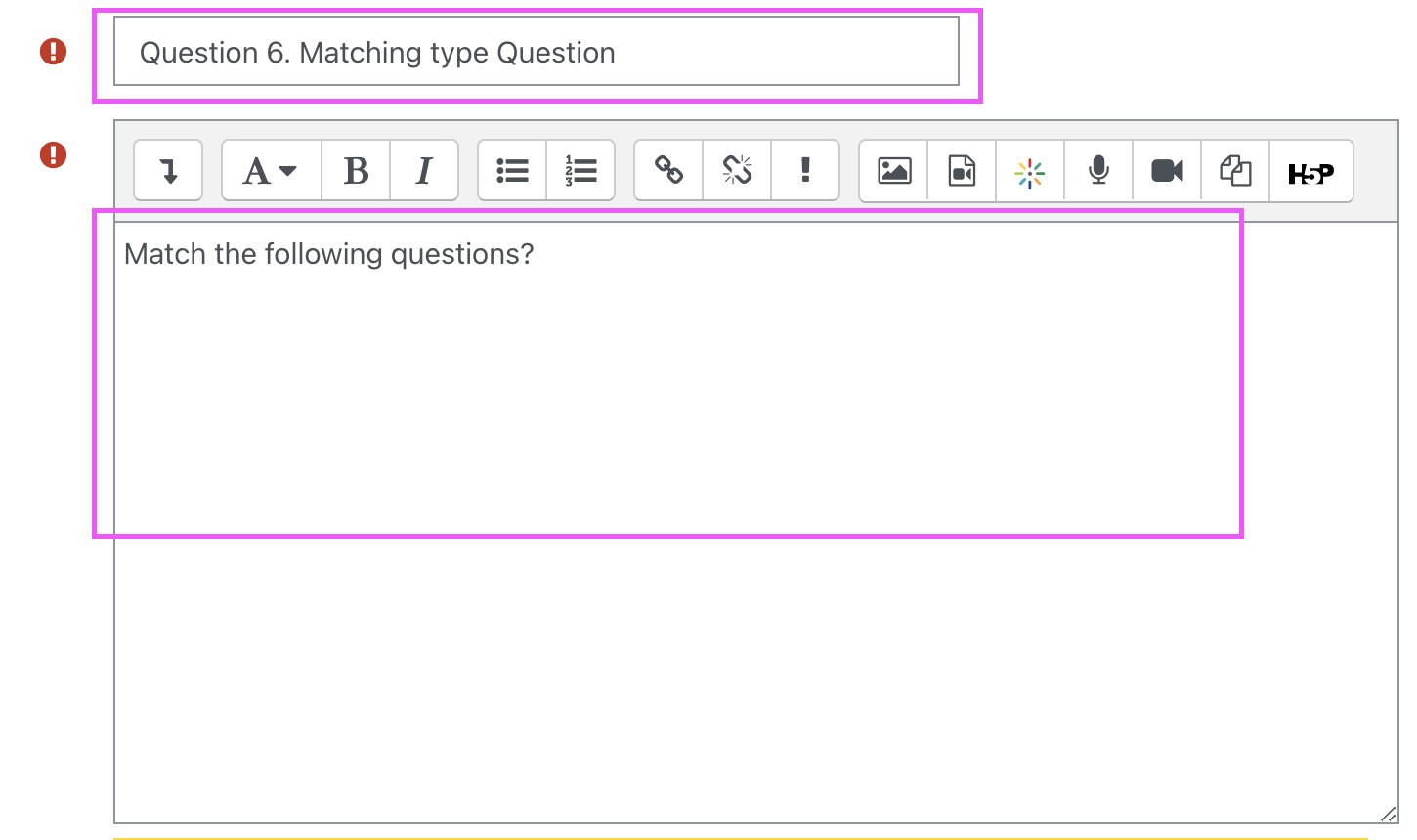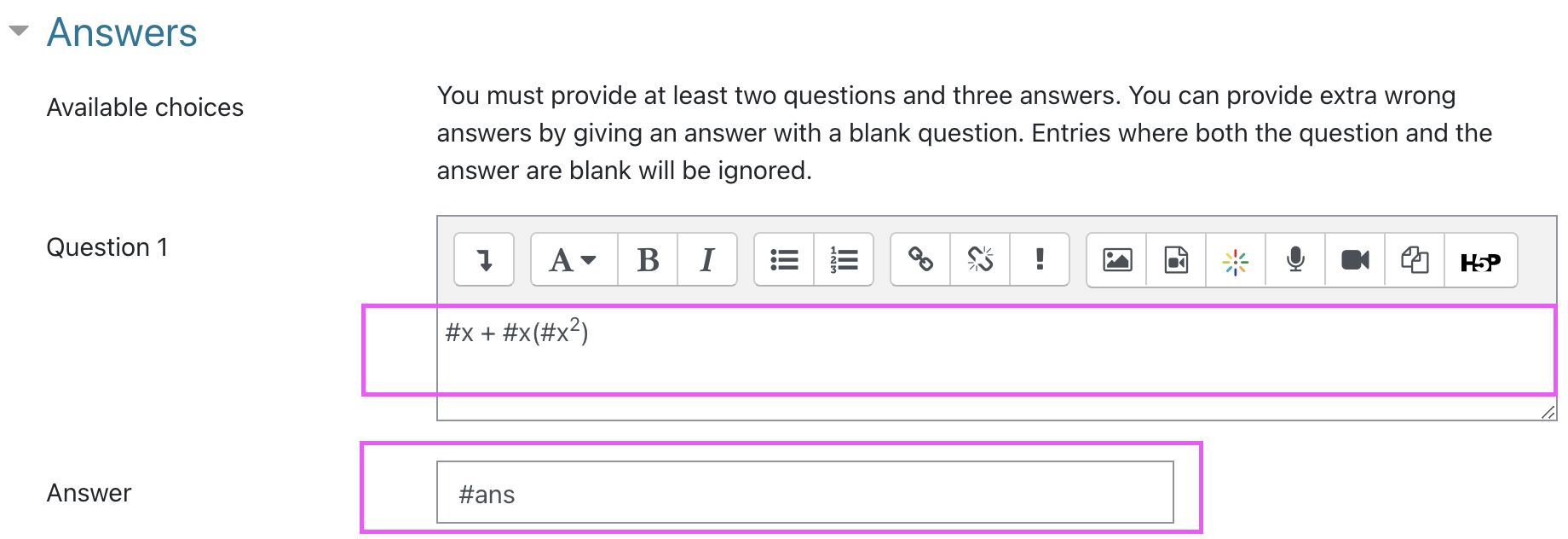In Question 2 we did not define any variables or formulas.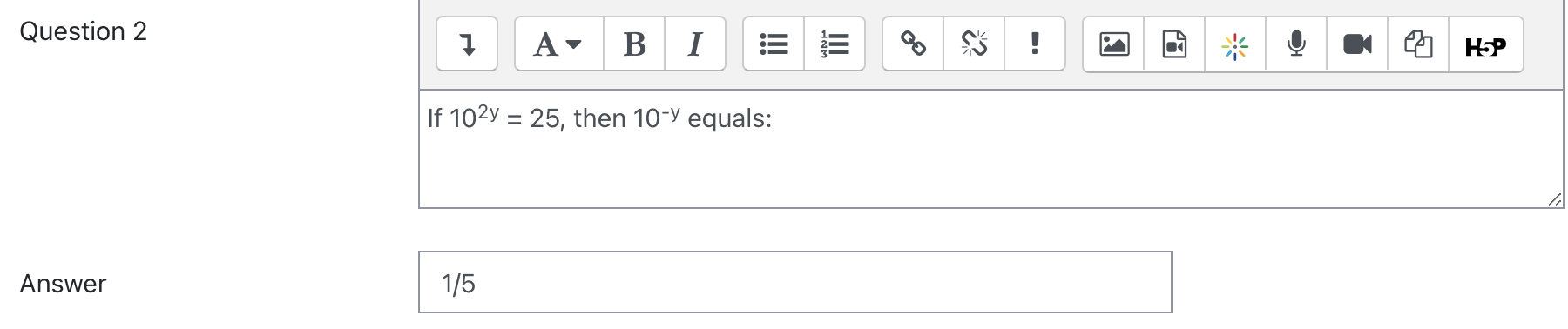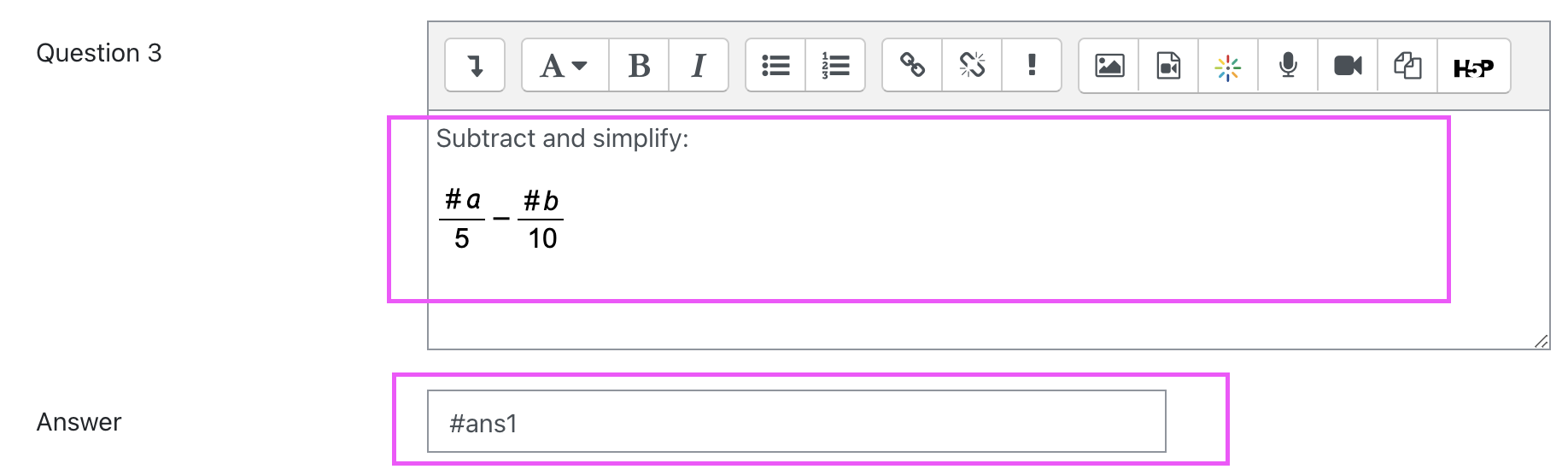Here is the example of this question

In the quiz settings goto the WIRIS variable option then click the gear icon to open the WIRISQuizzes StudioThen repeat the steps on question above on how to select your inputs, define your varible, formulas and function.

### The algorithm would be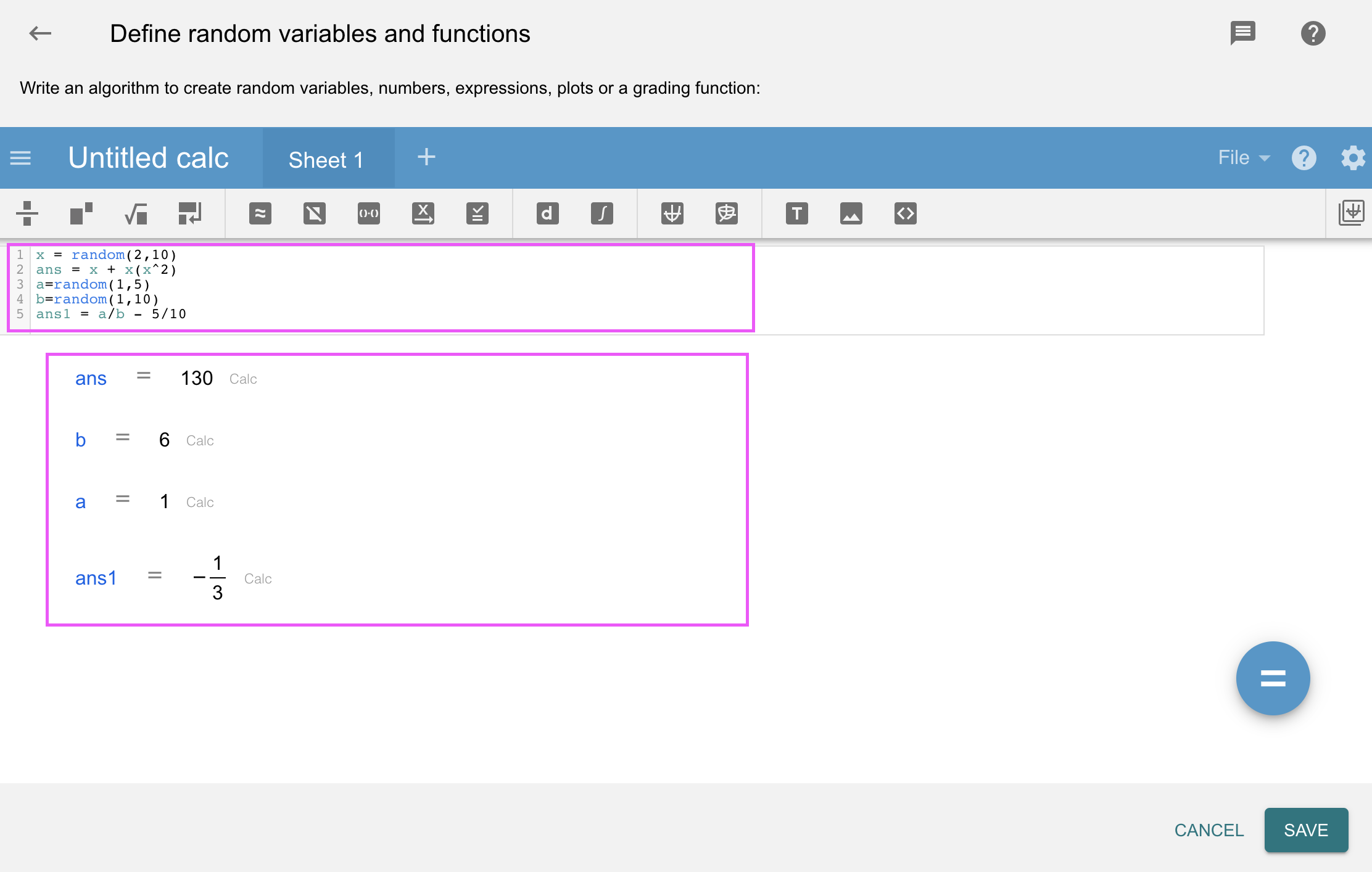### True or False

Students answer this question type by simply choosing between the two options true or false.

Define the variables you will use in the Question text and Feedback.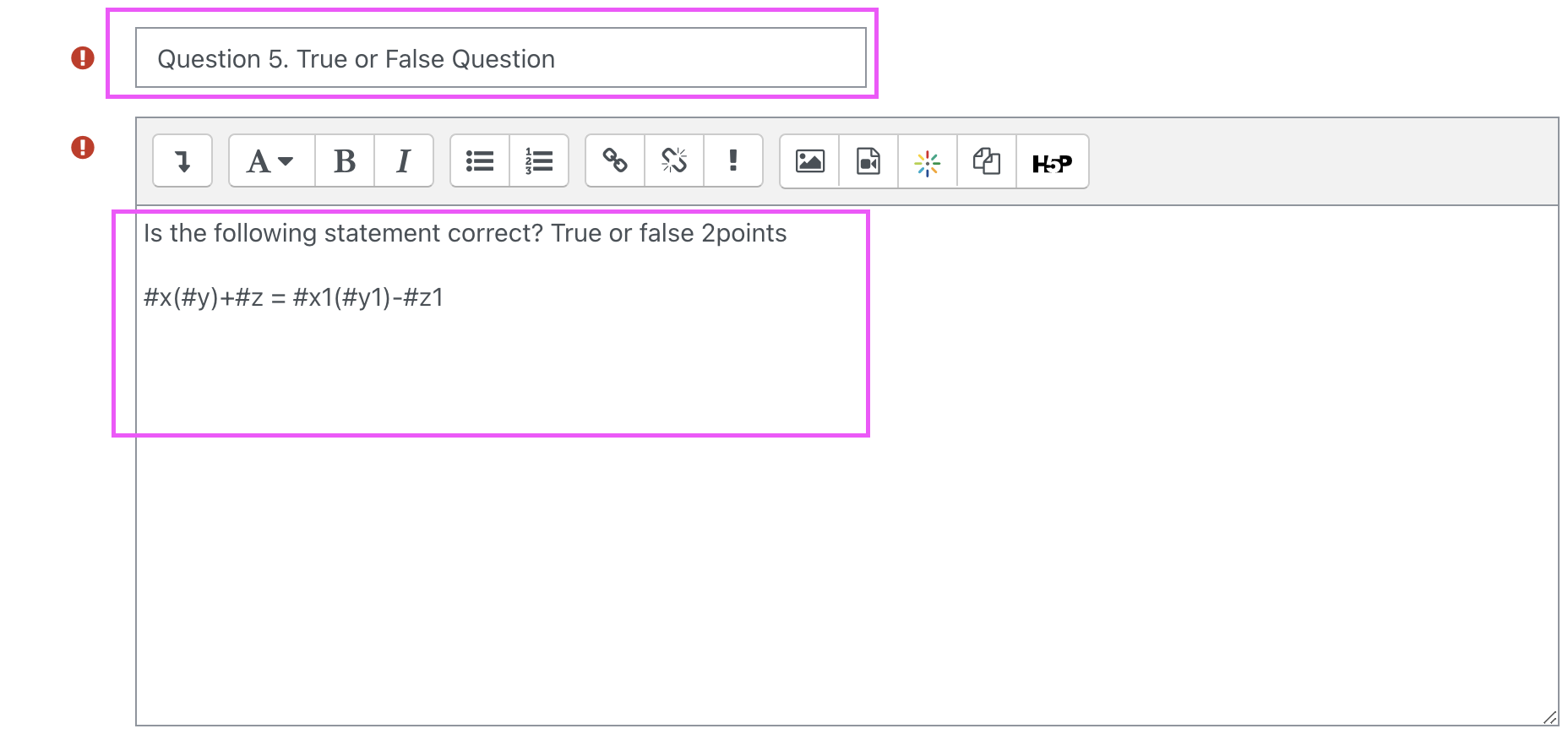### How to define Boolean Correct answer variable

If defined, this value will override the Correct answer field below. Write in the Variables tab an algorithm for setting this variable to "true" or "false".

Here we define varible #ans as the Boolean Correct answer.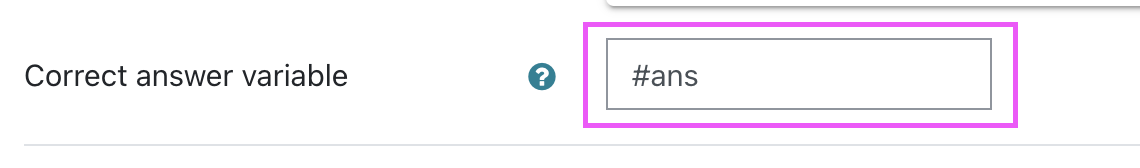To define the formula for the Boolean Correct answer

We had assign ans1 to get the value of x,y and z then ans2 for x1,y1 and z1

Then here is the formula to invoke the Boolean Correct answer ans = ans1 = ans2 where in the variable #ans will get the value of ans1 and ans2 if they are equal or not.

(if ans1 and ans2 is equal then Boolean Correct answer value will be True) or (if ans1 and ans2 is not equal then Boolean Correct answer value will be False)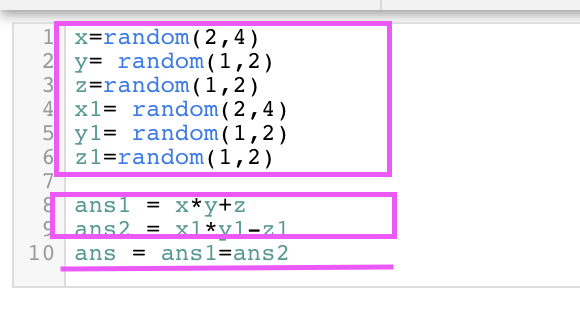### Testing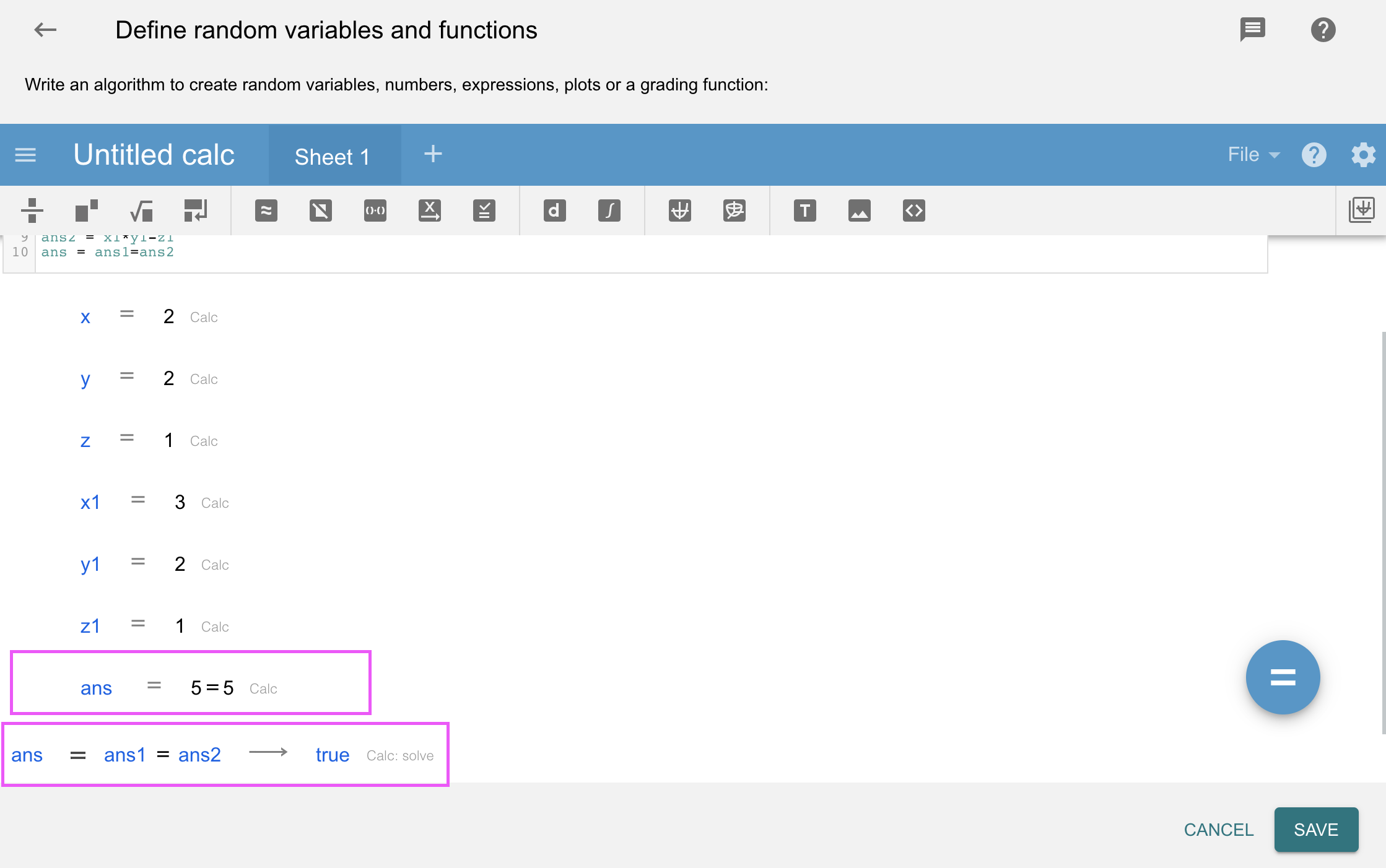### Cloze

Embedded answers, or cloze, allow inserting different question types in one question. For example: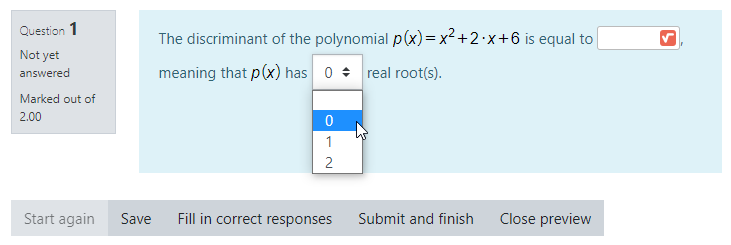In this example, there is a short answer type, as well as multiple choice. Detailed information on cloze questions can be found at Moodle docs. WIRIS cloze types follow the same exact format, with the following features:

There is no "Numerical" subtype because none is needed. Variables can be used, but the pound symbol "#" has to be escaped with a backslash (e.g. "#a" instead of the usual "#a"). The previous question, for example, could be made with the following text: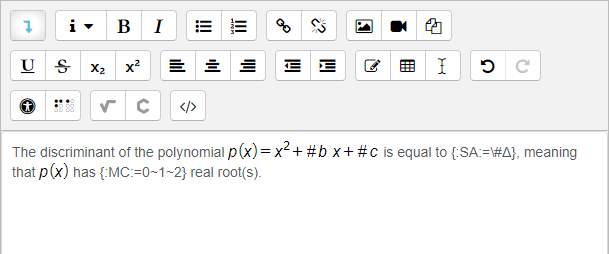and in the Define random variables and functions section: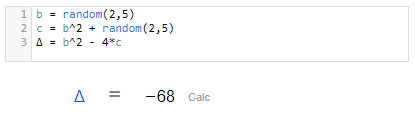In embedded short answer types, students can still write formulas in their answer with the editor popup by clicking on the square root symbol in the answer blank __?. To enable this, select "Math editor in a popup" in the Input options section: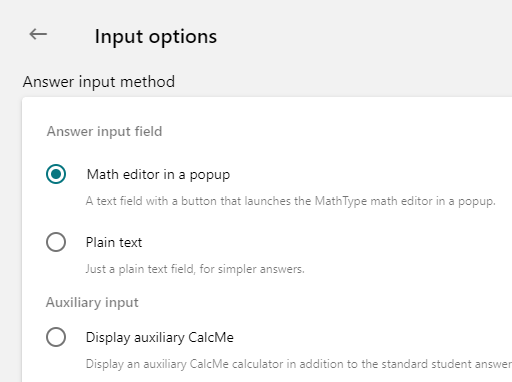Furthermore, we can notice that the algorithm of the question assures as that the polynomial will have no roots, so the question is not completely random. If we want to consider the three options, we have to change the answer format in order to add the new variables.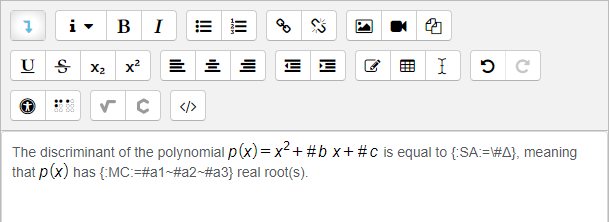Thus, the variable preceded by the sign = will be taken as the correct one and the others, those preceded by the sign ~, as the wrong ones. In the variables tab we will use: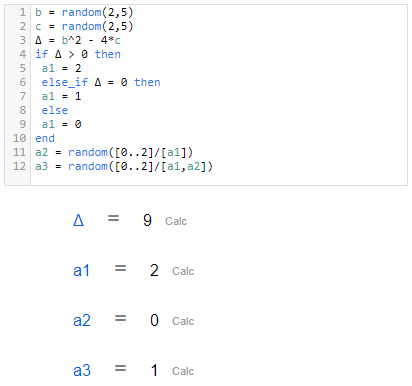As of Moodle 3.0, multichoice questions "MC" in cloze are not shuffled. Instead, there are shuffled multichoice types to accomplish this in cloze. These are:

Unshuffled Shuffled
Drop-down MC MCS
Horizontal MCH MCHS
Vertical MCV MCVS

The previous types are also available in the WIRIS cloze type. For example, if we replace the "MC" above with "MCHS" the question will look like: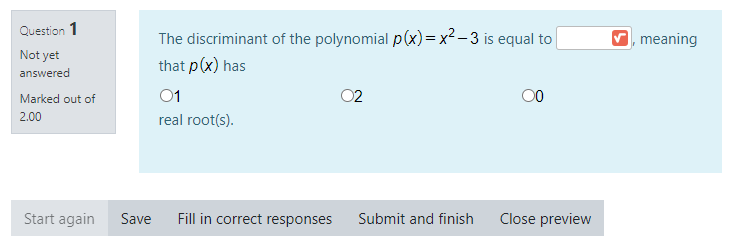## Text

Students can answer with plain text removing any mathematical comparison. For pure text answers with no mathematical content, you need to select the Text answer type.

https://docs.wiris.com/en/quizzes/user_interface/validation/text

## Lists and sets

You can have answers with lists and sets. There are multiple forms understood as lists; you can configure it in the Input options and Validation options sections.

https://docs.wiris.com/en/quizzes/user_interface/validation/lists_sets

## Automatic tables

It is possible to generate tables from variables created in our algorithm. The most basic feature is to create tables from lists. It is also possible to generate a table from a matrix. In the next sections, we explain both options.

https://docs.wiris.com/en/quizzes/user_interface/validation/tables

Now, Wiris Quizzes allows answers with subindices so you can ask even more questions. For instance, a common question about chemistry or symbolic maths is possible.

## Plus/Minus Operator

It is possible to perform computations with the plus-or-minus operator, as explained here. Therefore, the student is allowed to use the plus-or-minus symbol too. Recall that the result of the plus-or-minus operator is a list (not a set!).

## User functions

Wiris Quizzes Studio uses symbolic notation so we can work with variables and symbols. This is extremely useful but then f(x) is understood as a multiplication begin mathsize 22px style f times x end style. If we want that to be interpreted as a function begin mathsize 22px style f end style evaluated at a point begin mathsize 22px style x end style, we need to declare it as a User function in Input options > Input syntax > Define a new function.

https://docs.wiris.com/en/quizzes/user_interface/validation/user-functions

## Percentages and per mille

Students can use percent and per mille in the answers.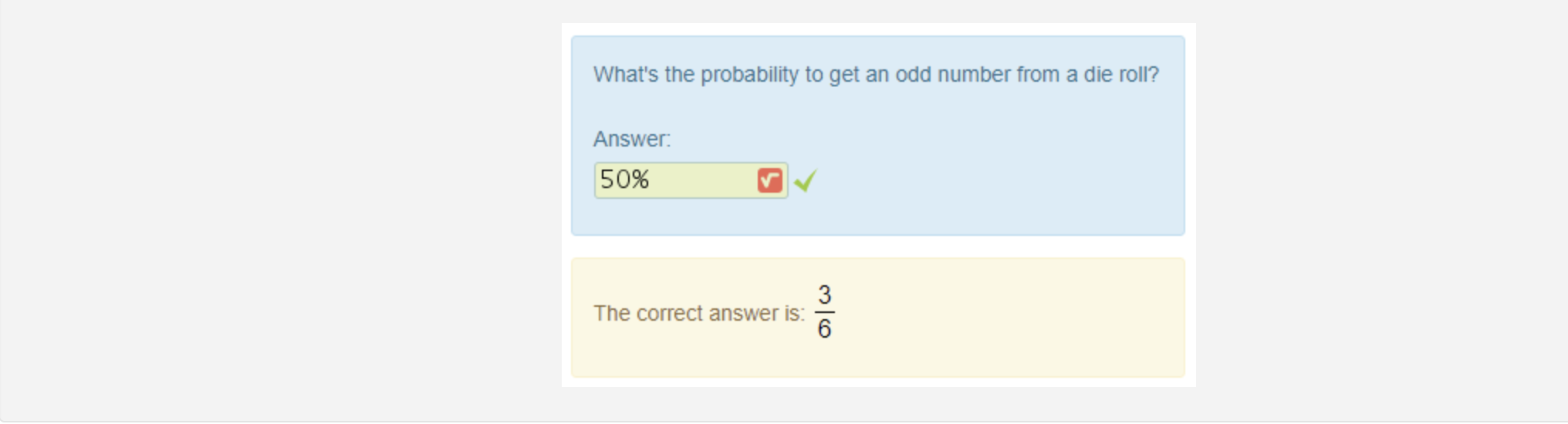The symbol percent (%) must be typed with the keyboard while the symbol per mille (‰) has a button in the answer toolbar. If you expect the students to answer with per mille, you must provide them the MathType toolbar. Alternatively, you can provide that symbol in the question wording for them to copy & paste. They can also use the combo ALT+0137 (numeric keypad).

https://docs.wiris.com/en/quizzes/user_interface/validation/percentages